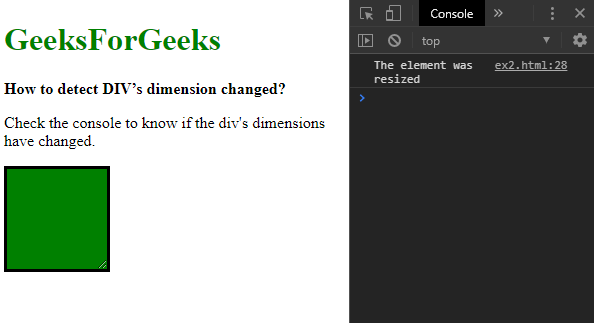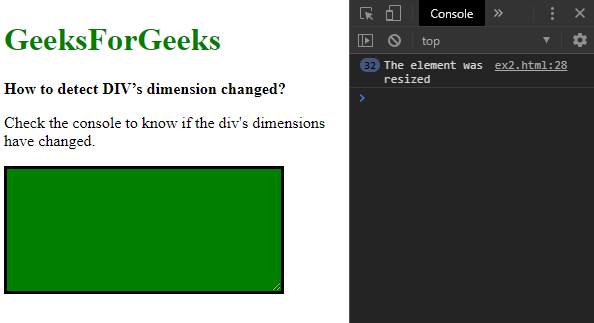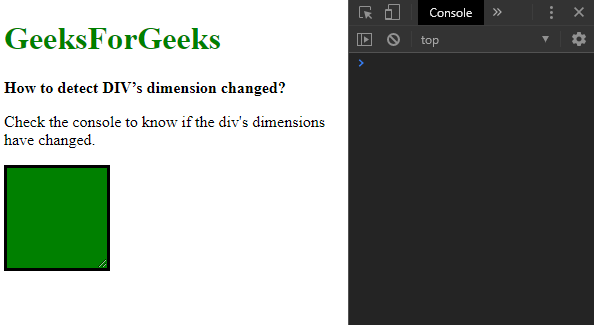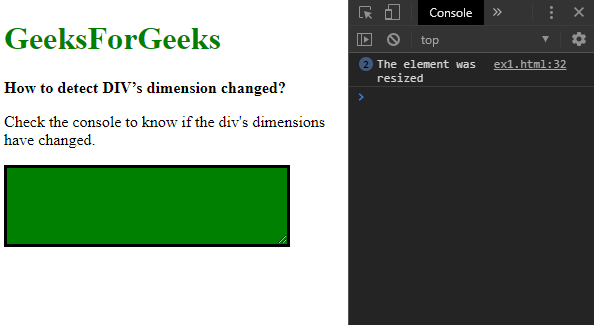# How to detect the change in DIV’s dimension ?

• Last Updated : 31 Oct, 2019

The change in a div’s dimension can be detected using 2 approaches:

Method 1: Checking for changes using the ResizeObserver Interface
The ResizeObserver Interface is used to report changes in dimensions of an element.
The element to be tracked is first selected using jQuery. A ResizeObserver object is created with a callback function that defines what action should be performed when a change in dimension is detected.
The observe() method of this object is used on the element that is to be tracked. This will check for any changes and execute the callback function when any dimension change is detected.

Syntax:

 `let resizeObserver = ``new` `ResizeObserver(() => {``    ``console.log(``"The element was resized"``);``});`` ` `resizeObserver.observe(elem);`

Example:

 ```<``html``>`` ` `<``head``>``    ``<``title``>``        ``How to detect DIV’s dimension changed?``    ````    ``<``style``>``        ``#box {``            ``resize: both;``            ``border: solid;``            ``background-color: green;``            ``height: 100px;``            ``width: 100px;``            ``overflow: auto;``        ``}``    `````` ` `<``body``>``    ``<``h1` `style``=``"color: green"``>``      ``GeeksForGeeks``    ````    ``<``b``>``      ``How to detect DIV’s dimension changed?``    ````    ``<``p``>Check the console to know if the div's dimensions have changed.``    ````    ``<``div` `id``=``"box"``>``    ``<``script` `src``=``"https://code.jquery.com/jquery-3.3.1.min.js"``>``    ```` ` `    ``<``script``>``        ``elem = \$("#box");`` ` `        ``let resizeObserver = new ResizeObserver(() => {``            ``console.log("The element was resized");``        ``});`` ` `        ``resizeObserver.observe(elem);``    `````` ` ``

Output:

• Before resizing the element:• After resizing the element:Method 2: Checking for changes in dimensions every 500 milliseconds
This method involves checking the dimensions of the element to be tracked every 500 milliseconds. The values of the dimensions are compared with the values of the older iteration to check if there is any difference.

The height and width of the element to be tracked is found out using the height() and width() method in jQuery. These dimensions will be used as the last tracked baseline dimensions of the element and stored in a variable.

A new function is created in which the element’s height and width are found out. This will be the new dimensions which will be compared with the previous ones. An if-statement is used where this new height and width are compared with the previously found baseline. If the dimensions do not match, it means that the dimensions have changed. The required action to be taken when dimensions change would be executed here.
The new dimensions found would be assigned to the older ones as a baseline so that the next iteration can be checked with them.
The function created is continuously in a loop every 500 milliseconds using the setInterval() function. This will continuously check for the changes in height and width and execute the given function whenever there is a difference.
This method is considerably slower than the previous one and decreasing the time between each check would further reduce the performance.

Syntax:

 `let lastHeight = \$(``"#box"``).height();``let lastWidth = \$(``"#box"``).width();`` ` `function` `checkHeightChange() {``    ``newHeight = \$(``"#box"``).height();``    ``newWidth = \$(``"#box"``).width();`` ` `    ``if` `(lastHeight != newHeight || lastWidth != newWidth) {``        ``console.log(``"The element was resized"``);`` ` `        ``// assign the new dimensions``        ``lastHeight = newHeight;``        ``lastWidth = newWidth;``     ``}`` ``}`` ` `setInterval(checkHeightChange, 500);`

Example:

 ```<``html``>`` ` `<``head``>``    ``<``title``>``        ``How to detect DIV’s dimension changed?``    ````    ``<``style``>``        ``#box {``            ``resize: both;``            ``border: solid;``            ``background-color: green;``            ``height: 100px;``            ``width: 100px;``            ``overflow: auto;``        ``}``    `````` ` `<``body``>``    ``<``h1` `style``=``"color: green"``>``      ``GeeksForGeeks``    ````    ``<``b``>``      ``How to detect DIV’s dimension changed?``    ````    ``<``p``>Check the console to know if the div's dimensions have changed.``    ````    ``<``div` `id``=``"box"``>``    ``<``script` `src``=``"https://code.jquery.com/jquery-3.3.1.min.js"``>``    ```` ` `    ``<``script` `type``=``"text/javascript"``>``        ``let lastHeight = \$("#box").height();``        ``let lastWidth = \$("#box").width();`` ` `        ``function checkHeightChange() {``            ``newHeight = \$("#box").height();``            ``newWidth = \$("#box").width();`` ` `            ``if (lastHeight != newHeight ||``                ``lastWidth != newWidth) {``                ``console.log("The element was resized");`` ` `                ``// assign the new dimensions``                ``lastHeight = newHeight;``                ``lastWidth = newWidth;``            ``}``        ``}`` ` `        ``setInterval(checkHeightChange, 500);``    `````` ` ``

Output:

• Before resizing the element:• After resizing the element:My Personal Notes arrow_drop_up